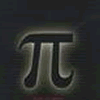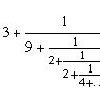Search by Topic

Resources tagged with Continued fractions similar to Cocked Hat:

Filter by: Content type:
Age range:
Challenge level:

There are 17 results

Broad Topics > Fractions, Decimals, Percentages, Ratio and Proportion > Continued fractionsGolden Mathematics

Age 16 to 18

A voyage of discovery through a sequence of challenges exploring properties of the Golden Ratio and Fibonacci numbers.Good Approximations

Age 16 to 18 Challenge Level:

Solve quadratic equations and use continued fractions to find rational approximations to irrational numbers.Continued Fractions I

Age 14 to 18

An article introducing continued fractions with some simple puzzles for the reader.Golden Fractions

Age 16 to 18 Challenge Level:

Find the link between a sequence of continued fractions and the ratio of succesive Fibonacci numbers.Continued Fractions II

Age 16 to 18

In this article we show that every whole number can be written as a continued fraction of the form k/(1+k/(1+k/...)).Infinite Continued Fractions

Age 16 to 18

In this article we are going to look at infinite continued fractions - continued fractions that do not terminate.The Golden Ratio, Fibonacci Numbers and Continued Fractions.

Age 14 to 16

An iterative method for finding the value of the Golden Ratio with explanations of how this involves the ratios of Fibonacci numbers and continued fractions.More Twisting and Turning

Age 11 to 16 Challenge Level:

It would be nice to have a strategy for disentangling any tangled ropes...All Tangled Up

Age 14 to 18 Challenge Level:

Can you tangle yourself up and reach any fraction?Comparing Continued Fractions

Age 16 to 18 Challenge Level:

Which of these continued fractions is bigger and why?Resistance

Age 16 to 18 Challenge Level:

Find the equation from which to calculate the resistance of an infinite network of resistances.Tangles

Age 11 to 16

A personal investigation of Conway's Rational Tangles. What were the interesting questions that needed to be asked, and where did they lead?There's a Limit

Age 14 to 18 Challenge Level:

Explore the continued fraction: 2+3/(2+3/(2+3/2+...)) What do you notice when successive terms are taken? What happens to the terms if the fraction goes on indefinitely?Approximations, Euclid's Algorithm & Continued Fractions

Age 16 to 18

This article sets some puzzles and describes how Euclid's algorithm and continued fractions are related.Not Continued Fractions

Age 14 to 18 Challenge Level:

Which rational numbers cannot be written in the form x + 1/(y + 1/z) where x, y and z are integers?Euclid's Algorithm and Musical Intervals

Age 16 to 18 Challenge Level:

Use Euclid's algorithm to get a rational approximation to the number of major thirds in an octave.Symmetric Tangles

Age 14 to 16

The tangles created by the twists and turns of the Conway rope trick are surprisingly symmetrical. Here's why!